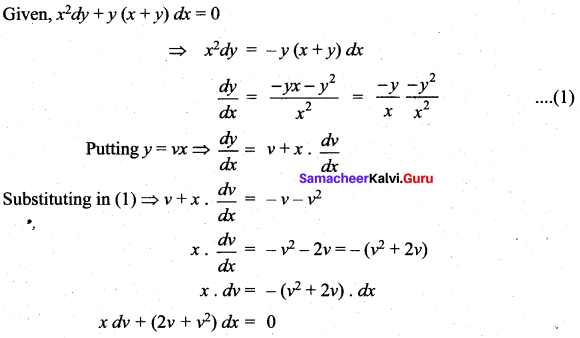# Samacheer Kalvi 12th Maths Solutions Chapter 10 Ordinary Differential Equations Ex 10.9

## Tamilnadu Samacheer Kalvi 12th Maths Solutions Chapter 10 Ordinary Differential Equations Ex 10.9

Choose the correct or the most suitable answer from the given four alternatives:

Question 1.
The order and degree of the differential equation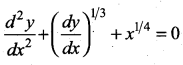are respectively ………
(a) 2, 3
(b) 3, 3
(c) 2, 6
(d) 2, 4
Solution:
(a) 2, 3
Hint: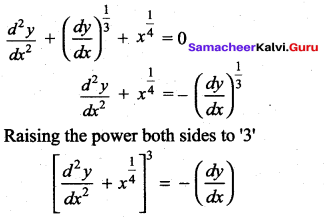Order = 2,
degree = 3

Question 2.
The differential equation representing the family of curves y = A cos (x + B), where A and B
are parameters, is …….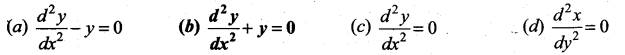Solution:
(b) $$\frac{d^{2} y}{d x^{2}}+y=0$$
Hint: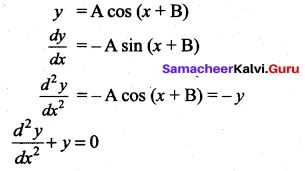Question 3.
The order and degree of the differential equation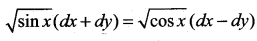is …..
(a) 1, 2
(b) 2, 2
(c) 1, 1
(d) 2, 1
Solution:
(c) 1, 1
Hint: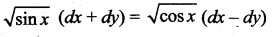Since, the first order derivatives are involved, Order = 1 and degree 1.

Question 4.
The order of the differential equation of all circles with centre at (h, k) and radius ‘a’ is …….
(a) 2
(b) 3
(c) 4
(d) 1
Solution:
(a) 2
Hint:
Equation of circle is (x – h)2 + (y – k)2 = a2
Equation is to be differentiated twice as two parameters are given.
∴ Order = 2

Question 5.
The differential equation of the family of curves y = Aex + Be-x, where A and B are arbitrary constants is ……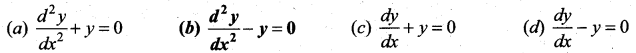Solution:
$$\frac{d^{2} y}{d x^{2}}-y=0$$
Hint: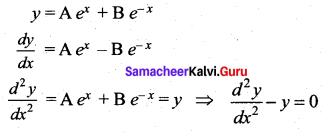Question 6.
The general solution of the differential equation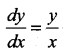is …..
(a) xy = k
(b) y = k log x
(c) y = kx
(d) log y = kx
Solution:
(c) y = kxQuestion 7.
The solution of the differential equation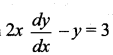represents ……..
(a) straight lines
(b) circles
(c) parabola
(d) ellipse
Solution:
(c) parabola
Hint: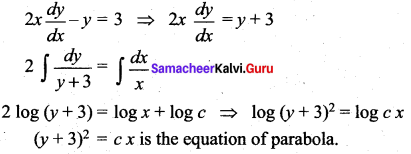Question 8.
The solution of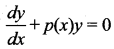is …….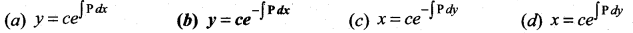Solution:
(b) $$y=c e^{-\int \mathbf{P} d x}$$
Hint: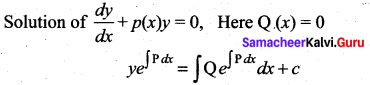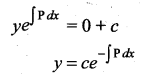Question 9.
The integrating factor of the differential equation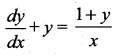is …….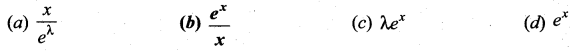Solution:
(b) $$\frac{e^{x}}{x}$$
Hint: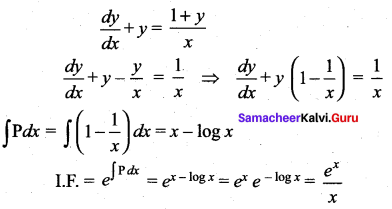Question 10.
The integrating factor of the differential equation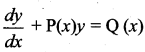is x, then P(x) …………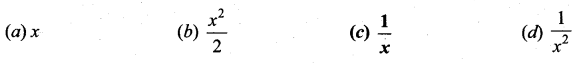Hint: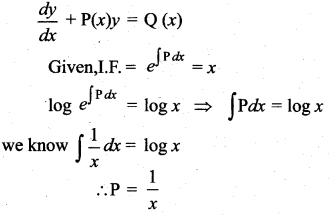Question 11.
The degree of the dififerential equation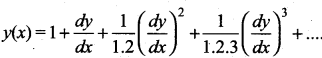is ……..
(a) 2
(b) 3
(c) 1
(d) 4
Solution:
(c) 1
Hint:
Degree = 1

Question 12.
If p and q are the order and degree of the differential equationwhen …..
(a) p < q
(b) p = q
(c) p > q
(d) p exists and q does not exist
Solution:
(c) p > q
Hint: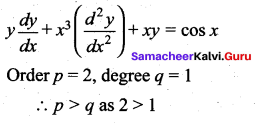Question 13.
The solution of the differential equation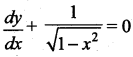is …….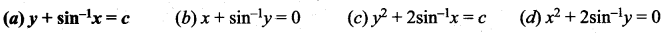Solution:
(a) $$y+\sin ^{-1} x=c$$
Hint: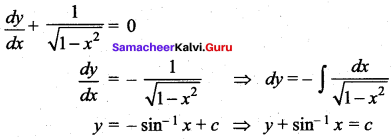Question 14.
The solution of the differential equation $$\frac{d y}{d x}=2 x y$$ is ………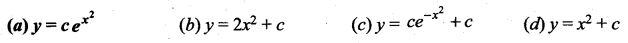Solution:
(a) $$y=c e^{x^{2}}$$
Hint: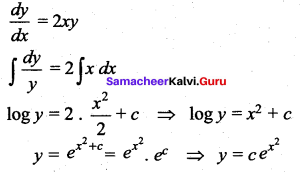Question 15.
The general solution of the differential equation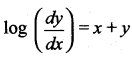is ……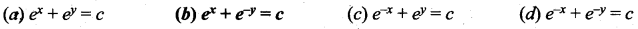Solution:
(b) $$e^{x}+e^{-y}=c$$
Hint: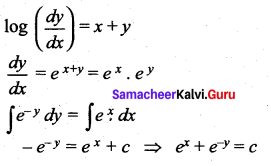Question 16.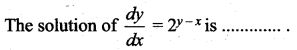Solution:
(c) $$\frac{1}{2^{x}}-\frac{1}{2^{y}}=c$$
Hint: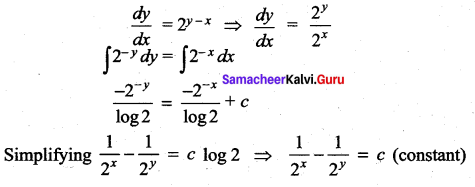Question 17.
The solution of the differential equation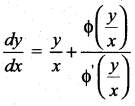is ……..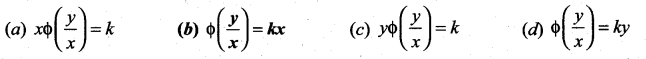Solution:
(b) $$\phi\left(\frac{y}{x}\right)=k x$$
Hint: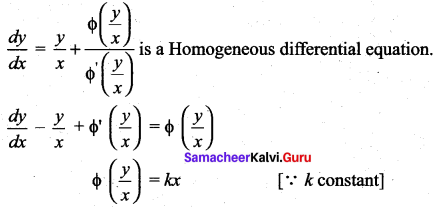Question 18.
If sin x is the integrating factor of the linear differential equation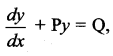, then P is ……
(a) log sin x
(b) cos x
(c) tan x
(d) cot x
Solution:
(d) cot x
Hint: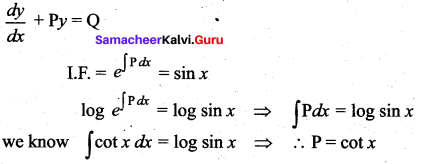Question 19.
The number of arbitrary constants in the general solutions of order n and n + 1 are respectively ……….
(a) n – 1, n
(b) n, n + 1
(c) n + 1, n + 2
(d) n + 1, n
Solution:
(b) n, n + 1

Question 20.
The number of arbitrary constants in the particular solution of a differential equation of third order is ………….
(a) 3
(b) 2
(c) 1
(d) 0
Solution:
(d) 0Question 21.
Integrating factor of the differential equation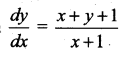is ……..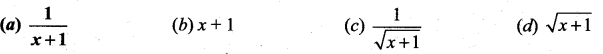Solution:
(a) $$\frac{1}{x+1}$$
Hint: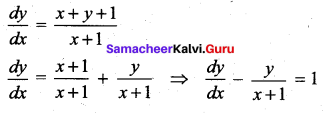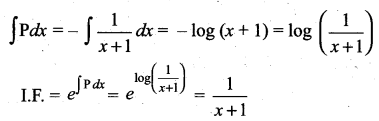Question 22.
The population P in any year t is such that the rate of increase in the population is proportional to the population. Then ……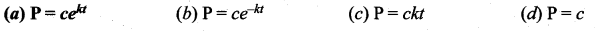Solution:
(a) $$\mathbf{P}=c e^{k t}$$
Hint: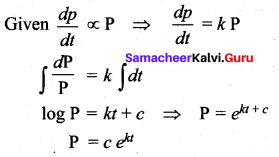Question 23.
P is the amount of certain substance left in after time t. If the rate of evaporation of the substance is proportional to the amount remaining, then ……
(a) P = cekt
(b) P = ce-kt
(c) P = ckt
(d) Pt = c
Solution:
(b) P = ce-kt
Hint: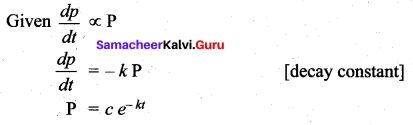Question 24.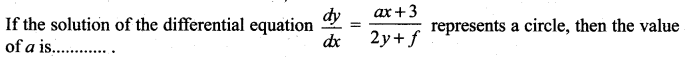(a) 2
(b) -2
(c )1
(d) -1
Solution:
(b) -2
Hint: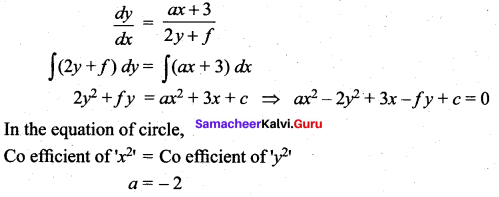Question 25.
The slope at any point of a curve y =f (x) is given by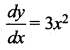and it passes through (-1, 1). Then the equation of the curve is ……..
(a) y = x3 + 2
(b) y = 3x2 + 4
(c) y = 3x3 + 4
(d) y = x3 + 5
Solution:
(a) y = x3 + 2
Hint: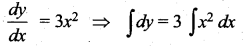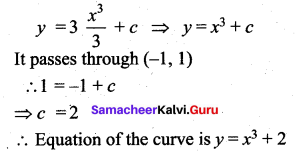### Samacheer Kalvi 12th Maths Solutions Chapter 10 Ordinary Differential Equations Ex 10.9 Additional Problems

Choose the correct or the most suitable answer from the given four alternatives:

Question 1.
The integrating factor of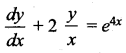is ………
(a) log x
(b) x2
(c) ex
(d) x
Solution:
(b) x2
Hint: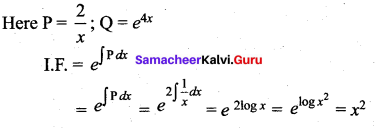Question 2.
If cos x is an integrating factor of the differential equation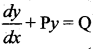then P = ……
(a) – cot x
(b) cot x
(c) tan x
(d) – tan x
Solution:
(d) – tan x

Question 3.
The integrating factor of dx + x dy = e-y sec2y dy is ……….
(a) ex
(b) e-x
(c) ey
(d) e-y
Solution:
(c) ey
Hint: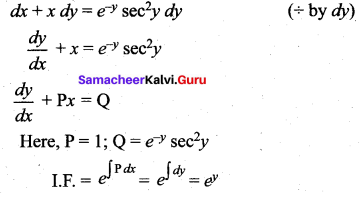Question 4.
Integrating factor of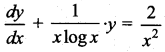is …..
(a) ex
(b) log x
(c) $$\frac{1}{x}$$
(d) e-x
Solution:
(b) log x
Hint: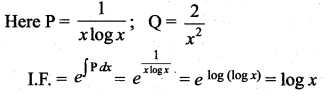Question 5.
Solution of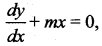where m < 0 is ……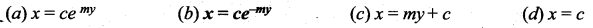Solution:
$$x=c e^{-m y}$$
Hint: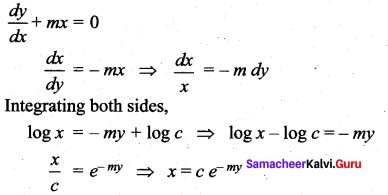Question 6.
y = cx – c2 is the general solution of the differential equation …….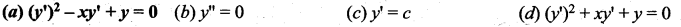Solution:
(a) $$\left(y^{\prime}\right)^{2}-x y^{\prime}+y=0$$
Hint: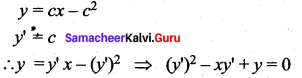Question 7.
The differential equation of all non-vertical lines in a plane is ……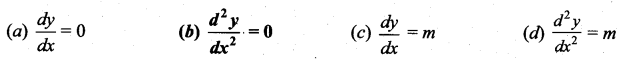Solution:
$$\frac{d^{2} y}{d x^{2}}=0$$
Hint:
The equation of the straight line is y = mx + c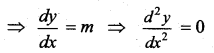Question 8.
The differential equation of all circles with centre at the origin is
(a) x dy + y dx = 0
(b) x dy – y dx = 0
(c) x dx + y dy = 0
(d) x dx – y dy = 0
Solution:
(c) x dx + y dy = 0
Hint:
The equation of family of circle with the centre at the origin is x2 + y2 = a2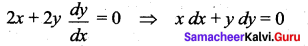Question 9.
The differential equation of the family of lines y = mx is ……..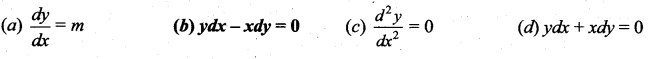Solution:
(d) 6
Hint: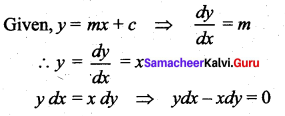Question 10.
The degree of the differential equation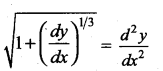(a) 1
(b) 2
(c) 3
(d) 6
Solution:
(d) 6
Hint: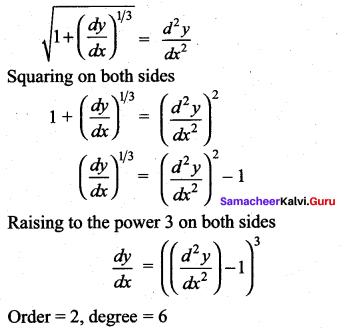Question 11.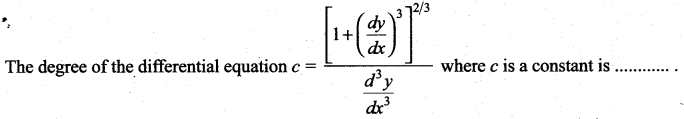(a) 1
(b) 3
(c) -2
(d) 2
Solution:
(b) 3
Hint: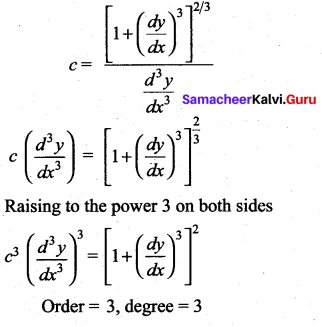Question 12.
The amount present in a radio active element disintegrates at a rate proportional to its amount. The differential equation corresponding to the above statement is (k is negative)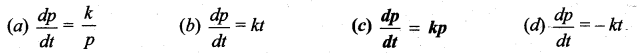Solution:
(c) $$\frac{d p}{d t}=k p$$
Hint:
Let p be the amount present in a radio active element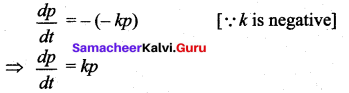Question 13.
On putting y = vx, the homogeneous differential equation x2dy + y (x + y)dx = 0 becomes …….
(a) xdv + (2v + v2) dx = 0
(b) vdx + (2x + x2)dv = 0
(c) v2dx – (x + x2) dv = 0
(d) vdv + (2x + x2) dx = 0
Solution:
(a) xdv + (2v + v2) dx = 0
Hint: